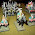## Thursday, 20 September 2012

### Probability for Wargamers 1 - Hitting on a 3This is going to be an occasional series on probability and statistics, and is largely going to set out to answer variants of the question 'just HOW hard was that?' or 'how ridiculously unlucky have I just been?'

For the first article, we'll go back to any number of games of WAB where I've demonstrated a prodigious ability to miss with the fair Cartimandua, Queen of the Brigantes. This one's quite useful, because it covers both of the two basic concepts of probability, namely when to add probabilities and when to multiply them.

So, to hit in WAB you need to roll equal to or greater than a certain number on a d6. If we want to get three or more, say, then four possible numbers on the dice will get us that: 3, 4, 5 or 6. Each of these has a 1/6 chance of coming up assuming the dice aren't loaded, and since these are mutually exclusive events (we can't roll a 3 AND a 6 on the same roll of one dice, for example), we can add the probabilities, which gives us a 4/6 chance, or 66.67%, of rolling 4 or less.

What's the odds of failing?

That's easy. We know that success and failure are a) mutually exclusive events and b) the only possible outcomes, so the total probability for them must add up to 1, or 100%. Hence the odds on failing are 100% minus the odds of success, i.e. 2/6, 1/3, or 33.33%. (Yes, obviously it's the 1/6 chance of rolling a 1 plus the 1/6 chance of rolling a 2, but sometimes you'll find this trick much easier.)

So: what's the chance of my barbarian Queen, with three attacks on a 3+, not hitting at all? That means I have to fail with the first dice, AND the second, AND the third. That phrasing is a dead giveaway - these are independent events (whatever your superstitions about dice), and this means that in order to figure out the odds of them all happening, the rules say we can multiply the probabilities together. So, the odds of failing three times are 1/3 * 1/3 * 1/3, or 1/27, which works out at 3.70%.

Man, my dice rolling sucks!

1.I find this a pain in Spearhead (for example). You usually need a 5 or 6 to hit (or occasionally an 11 or 12 on 2d6).

I'd prefer to just roll a number of d6 and count up the hits but Spearhead requires that each 'combat' is done individually.

So I have to roll one d6 each time - invariably falling foul of the stats you've described and having one hit for several attempts.

We keep threatening to document the dice throws during a game as one of our group (Russ) seems to deliver an inordinate number of 6's during a game. Perhaps we can put your stats to the test.

1.Although it makes no difference to the odds whether you roll 3 dice in one go and count the hits, or 3 dice one after the other.

The only advantage of rolling three dice one after the other, if you only need ONE hit, is that you can stop once you've succeeded and (in some systems) use the leftover dice for something else.

2.Try to use the main of the three dice. That will give more chances of hitting. Gauss curves and the like.

1.Sorry - not following you.

3.Sorry, my english is not enough for mathematical questions. If you throw the three dice and add the numbers, then divide by three, that is the average?, mean?. Doing this, you obtain less "ones" and less "sixes", and more average numbers. But you have to change the "to hit" tables. The distribution is a gauss curve:
http://en.wikipedia.org/wiki/Normal_distribution

1.This adding and dividing will give something more approximating a normal curve because a total of 10 or 11 (combinations of 6+4+1, 6+3+2 etc) on the three dice is much greater than a total of 3 (three ones) or 18 (three sixes).

The problem is, though: what sort of 'normal' - a flat one with a low mean and a large standard deviation; or a large mean and small standard deviation. That is, a broad flat hill, or a narrow steep mountain. As it happens 3D6/3 will give you the latter. The result probabilities go 1: 4/216; 2:31/216; 3:73/216; 4:73/216; 5:31/216; 6:4/216. This is pretty close to an average dice, except that on rare occasions, maybe once or twice in a whole battle, a 1 or a 6 will turn up.

But whether this 'shape' of 'normal curve' is acceptable will depend upon your rules for resolving combat. For instance (a simple example), if you were going for a straight 1D6 vs 1D6 'High roll wins' this might not be a good system as draws would be too frequent. Consider, too the situation in which you need 'x' to hit. If you need 5+, hits will be hard to come by (a 6 will occur so rarely as scarcely to be hoped for). A 4+ will be the usual 50-50 proposition; but if you need 3+, then at over 85% chance of success, you are going to feel unlucky if you miss.

Views and opinions expressed here are those of the commenter, not mine. I reserve the right to delete comments if I consider them unacceptable. Unfortunately due to persistent spam from one source, I've been forced to turn on captchas for comments.

Comments on posts older than 7 days will go into a moderation queue.